# The Shortest Route Problem[Page 274 ( continued )]

The shortest route problem is to determine the shortest distance between an originating point and several destination points. For example, the Stagecoach Shipping Company transports oranges by six trucks from Los Angeles to six cities in the West and Midwest. The different routes between Los Angeles and the destination cities and the length of time, in hours, required by a truck to travel each route are shown in Figure 7.2.

##### Figure 7.2. Shipping routes from Los Angeles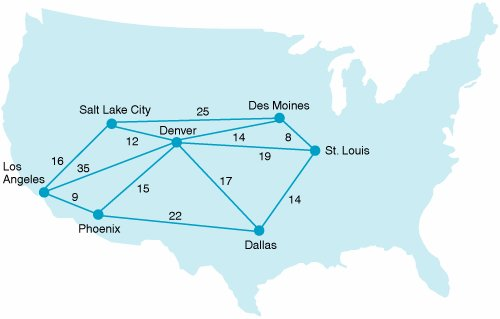The shortest route problem is to find the shortest distance between an origin and various destination points .

The shipping company manager wants to determine the best routes (in terms of the minimum travel time) for the trucks to take to reach their destinations. This problem can be solved by using the shortest route solution technique. In applying this technique, it is convenient to represent the system of truck routes as a network, as shown in Figure 7.3.

##### Figure 7.3. Network of shipping routes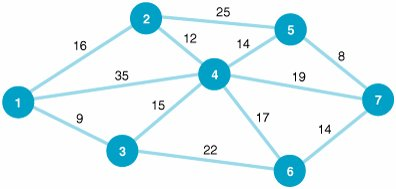To repeat our objective as it relates to Figure 7.3, we want to determine the shortest routes from the origin (node 1) to the six destinations (nodes 2 through 7).

[Page 275]

#### The Shortest Route Solution Approach

We begin the shortest route solution technique by starting at node 1 (the origin) and determining the shortest time required to get to a directly connected (i.e., adjacent) node. The three nodes directly connected to node 1 are 2, 3, and 4, as shown in Figure 7.4. Of these three nodes, the shortest time is 9 hours to node 3. Thus, we have determined our first shortest route from nodes 1 to 3 (i.e., from Los Angeles to Phoenix). We will now refer to nodes 1 and 3 as the permanent set to indicate that we have found the shortest route to these nodes. (Because node 1 has no route to it, it is automatically in the permanent set.)

##### Figure 7.4. Network with node 1 in the permanent set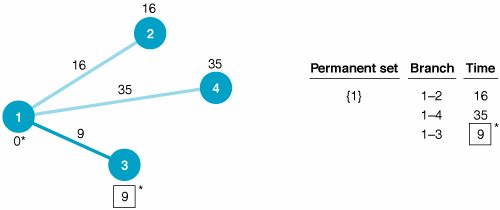Determine the initial shortest route from the origin (node 1) to the closest node (3) .

Notice in Figure 7.4 that the shortest route to node 3 is drawn with a heavy line, and the shortest time to node 3 (9 hours) is enclosed by a box. The table accompanying Figure 7.4 describes the process of selecting the shortest route. The permanent set is shown to contain only node 1. The three branches from node 1 are 12, 14, and 13, and this last branch has the minimum time of 9 hours.

Next , we will repeat the foregoing steps used to determine the shortest route to node 3. First, we must determine all the nodes directly connected to the nodes in the permanent set (nodes 1 and 3). Nodes 2, 4, and 6 are all directly connected to nodes 1 and 3, as shown in Figure 7.5.

##### Figure 7.5. Network with nodes 1 and 3 in the permanent set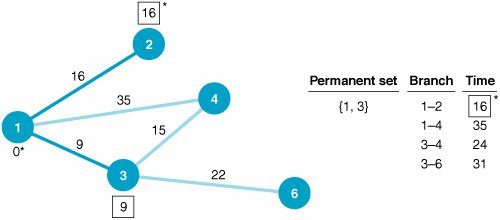Determine all nodes directly connected to the permanent set .

The next step is to determine the shortest route to the three nodes (2, 4, and 6) directly connected to the permanent set nodes. There are two branches starting from node 1 (12 and 14) and two branches from node 3 (34 and 36). The branch with the shortest time is to node 2, with a time of 16 hours. Thus, node 2 becomes part of the permanent set. Notice in our computations accompanying Figure 7.5 that the time to node 6 (branch 36) is 31 hours, which is determined by adding the branch 36 time of 22 hours to the shortest route time of 9 hours at node 3.

[Page 276]

As we move to the next step, the permanent set consists of nodes 1, 2, and 3. This indicates that we have found the shortest route to nodes 1, 2, and 3. We must now determine which nodes are directly connected to the permanent set nodes. Node 5 is the only adjacent node not presently connected to the permanent set, so it is connected directly to node 2. In addition, node 4 is now connected directly to node 2 (because node 2 has joined the permanent set). These additions are shown in Figure 7.6.

##### Figure 7.6. Network with nodes 1, 2, and 3 in the permanent set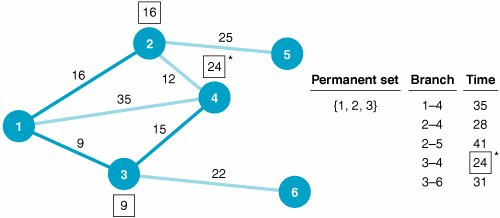Redefine the permanent set.

Five branches lead from the permanent set nodes (1, 2, and 3) to their directly connected nodes, as shown in the table accompanying Figure 7.6. The branch representing the route with the shortest time is 34, with a time of 24 hours. Thus, we have determined the shortest route to node 4, and it joins the permanent set. Notice that the shortest time to node 4 (24 hours) is the route from node 1 through node 3. The other routes to node 4 from node 1 through node 2 are longer; therefore, we will not consider them any further as possible routes to node 4.

To summarize, the shortest routes to nodes 1, 2, 3, and 4 have all been determined, and these nodes now form the permanent set.

Next, we repeat the process of determining the nodes directly connected to the permanent set nodes. These directly connected nodes are 5, 6, and 7, as shown in Figure 7.7. Notice in Figure 7.7 that we have eliminated the branches from nodes 1 and 2 to node 4 because we determined that the route with the shortest time to node 4 does not include these branches.

##### Figure 7.7. Network with nodes 1, 2, 3, and 4 in the permanent set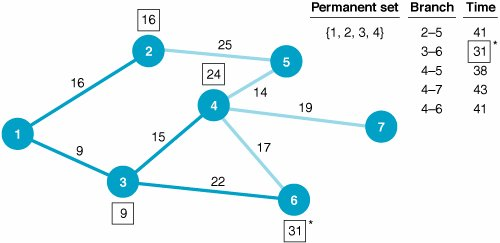[Page 277]

From the table accompanying Figure 7.7 we can see that of the branches leading to nodes 5, 6, and 7, branch 36 has the shortest cumulative time, of 31 hours. Thus, node 6 is added to our permanent set. This means that we have now found the shortest routes to nodes 1, 2, 3, 4, and 6.

Repeating the process, we observe that the nodes directly connected (adjacent) to our permanent set are nodes 5 and 7, as shown in Figure 7.8. (Notice that branch 46 has been eliminated because the best route to node 6 goes through node 3 instead of node 4.)

##### Figure 7.8. Network with nodes 1, 2, 3, 4, and 6 in the permanent set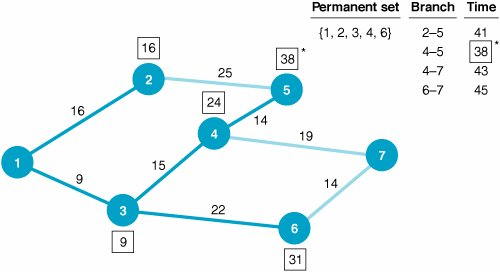Of the branches leading from the permanent set nodes to nodes 5 and 7, branch 45 has the shortest cumulative time, of 38 hours. Thus, node 5 joins the permanent set. We have now determined the routes with the shortest times to nodes 1, 2, 3, 4, 5, and 6 (as denoted by the heavy branches in Figure 7.8).

The only remaining node directly connected to the permanent set is node 7, as shown in Figure 7.9. Of the three branches connecting node 7 to the permanent set, branch 47 has the shortest time, of 43 hours. Therefore, node 7 joins the permanent set.

##### Figure 7.9. Network with nodes 1, 2, 3, 4, 5, and 6 in the permanent set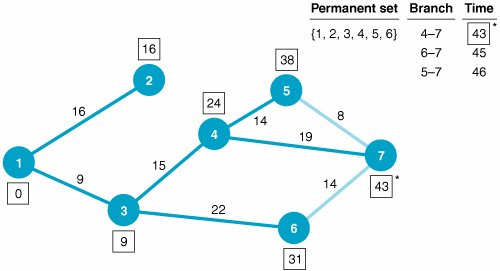The routes with the shortest times from the origin (node 1) to each of the other six nodes and their corresponding travel times are summarized in Figure 7.10 and Table 7.1.

[Page 278]
##### Figure 7.10. Network with optimal routes from Los Angeles to all destinations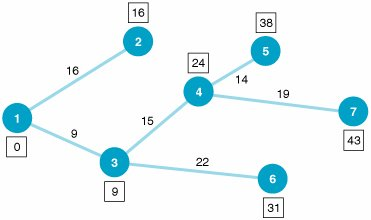##### Table 7.1. Shortest travel time from origin to each destination

From Los Angeles to:

Route

Total Hours

Salt Lake City (node 2)

12

16

Phoenix (node 3)

13

9

Denver (node 4)

134

24

Des Moines (node 5)

1345

38

Dallas (node 6)

136

31

St. Louis (node 7)

1347

43

In summary, the steps of the shortest route solution method are as follows :

1. Select the node with the shortest direct route from the origin.

2. Establish a permanent set with the origin node and the node that was selected in step 1.

3. Determine all nodes directly connected to the permanent set nodes.

4. Select the node with the shortest route (branch) from the group of nodes directly connected to the permanent set nodes.

5. Repeat steps 3 and 4 until all nodes have joined the permanent set.

Steps of the shortest route solution method .

#### Computer Solution of the Shortest Route Problem with QM for Windows

QM for Windows includes modules for each of the three network flow models that will be presented in this chaptershortest route, minimal spanning tree, and maximal flow. The QM for Windows solution for the shortest route from node 1 to node 7 for the Stagecoach Shipping Company example is shown in Exhibit 7.1.

##### Exhibit 7.1.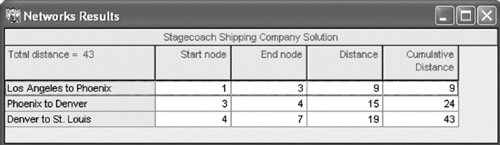[Page 279]

The solution screen in Exhibit 7.1 provides the shortest route from the start node, 1 (Los Angeles), to the end node, 7 (St. Louis). The shortest route from any node in the network to any other node can be determined by indicating the desired origin and destination nodes at the top of the solution screen. For example, the shortest route from node 1 to node 5 is shown in Exhibit 7.2.

##### Exhibit 7.2.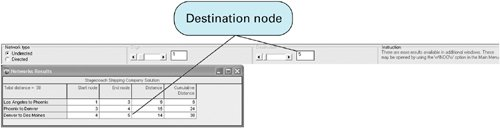#### Computer Solution of the Shortest Route Problem with Excel

We can also solve the shortest route problem with Excel spreadsheets by formulating and solving the shortest route network as a 01 integer linear programming problem. To formulate the linear programming model, we first define a decision variable for each branch in the network, as follows:

x ij = 0 if branch ij is not selected as part of the shortest route and 1 if branch ij is selected

To reduce the complexity and size of our model formulation, we will also assume that items can flow only from a lower node number to a higher node number (e.g., 3 to 4 but not 4 to 3). This greatly reduces the number of variables and branches to consider.

The objective function is formulated by minimizing the sum of the products of each branch value times the travel time for each branch:

minimize Z = 16 x 12 + 9 x 13 + 35 x 14 + 12 x 24 + 25 x 25 + 15 x 34 + 22 x 36 + 14 x 45 + 17 x 46 + 19 x 47 + 8 x 57 + 14 x 67

There is one constraint for each node, indicating that whatever comes into a node must also go out. This is referred to as conservation of flow . This means that one "truck" leaves node 1 (Los Angeles) either through branch 12, branch 13, or branch 14. This constraint for node 1 is formulated as

x 12 + x 13 + x 14 = 1

At node 2, a truck would come in through branch 12 and depart through 24 or 25, as follows:

x 12 = x 24 + x 25

This constraint can be rewritten as

x 12 x 24 x 25 = 0

[Page 280]

Constraints at nodes 3, 4, 5, 6, and 7 are constructed similarly, resulting in the complete linear programming model, summarized as follows: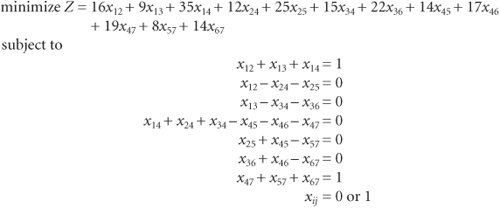This model is solved in Excel much the same way as any other linear programming problem, using the "Solver" option from the "Tools" menu at the top of the spreadsheet. Exhibit 7.3 shows an Excel spreadsheet set up to solve the Stagecoach Shipping Company example. The decision variables, x ij , are represented by cells A6:A17 . Thus, a value of 1 in one of these cells means that branch has been selected as part of the shortest route. Cells F6:F17 contain the travel times (in hours) for each branch, and the objective function formula is contained in cell F18, shown on the formula bar at the top of the screen. The model constraints reflecting the flow through each node are included in the box on the right side of the spreadsheet. For example, cell I6 contains the constraint formula for node 1, =A6+A7+A8 , and cell I7 contains the constraint formula for node 2, =A6-A9-A10 .

##### Exhibit 7.3.Exhibit 7.4 shows the Solver screen, which is accessed from the "Tools" menu at the top of the screen. Notice that the constraint for node 1 embedded in cell I6 equals 1, indicating that one truck is leaving node 1, and the constraint for node 7, embedded in cell I12, also equals 1, indicating that one truck will end up at node 7.

[Page 281]
##### Exhibit 7.4.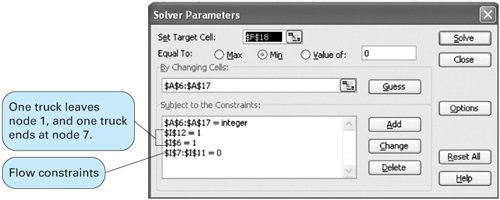The Excel solution is shown in Exhibit 7.5. Notice that cells A7, A11, and A15 have values of 1 in them, indicating that these branches, 13, 34, and 47, are on the shortest route. The total distance in travel time is 43 hours, as shown in cell F18.

##### Exhibit 7.5.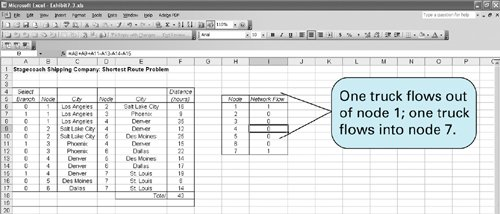Introduction to Management Science (10th Edition)
ISBN: 0136064361
EAN: 2147483647
Year: 2006
Pages: 358

Similar book on Amazon